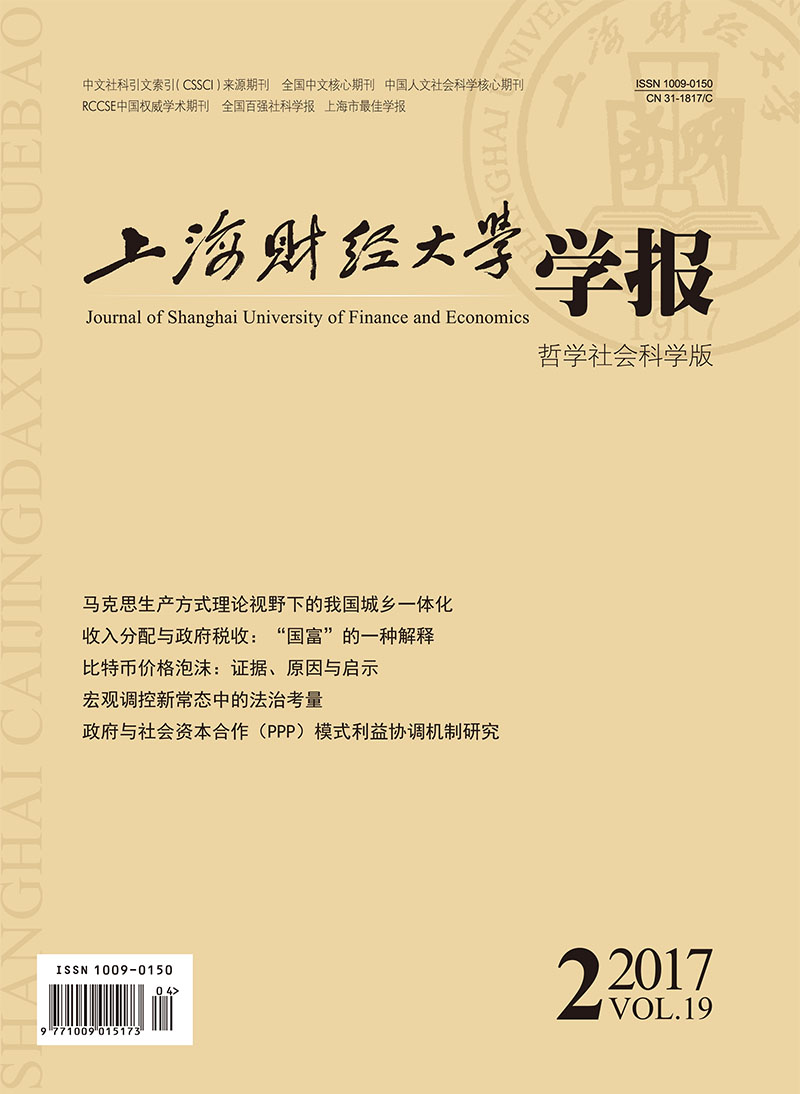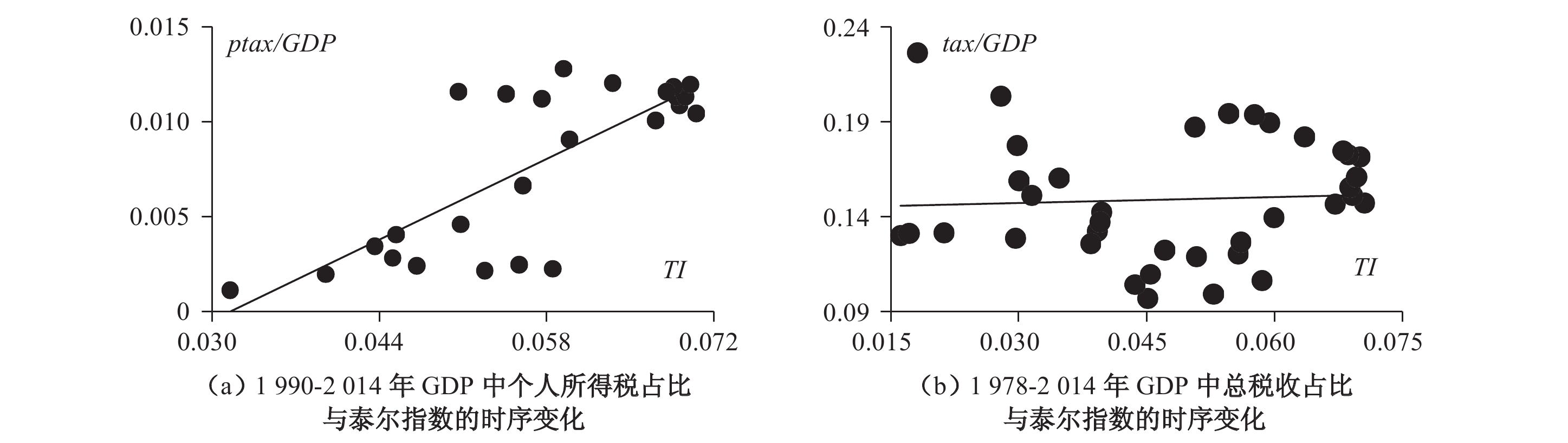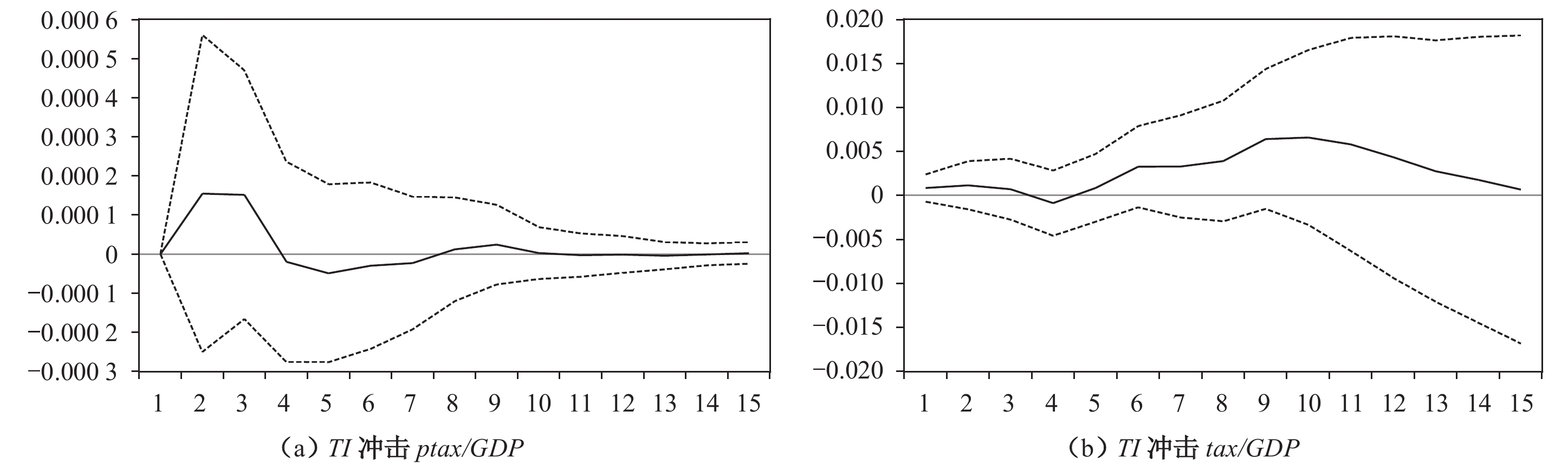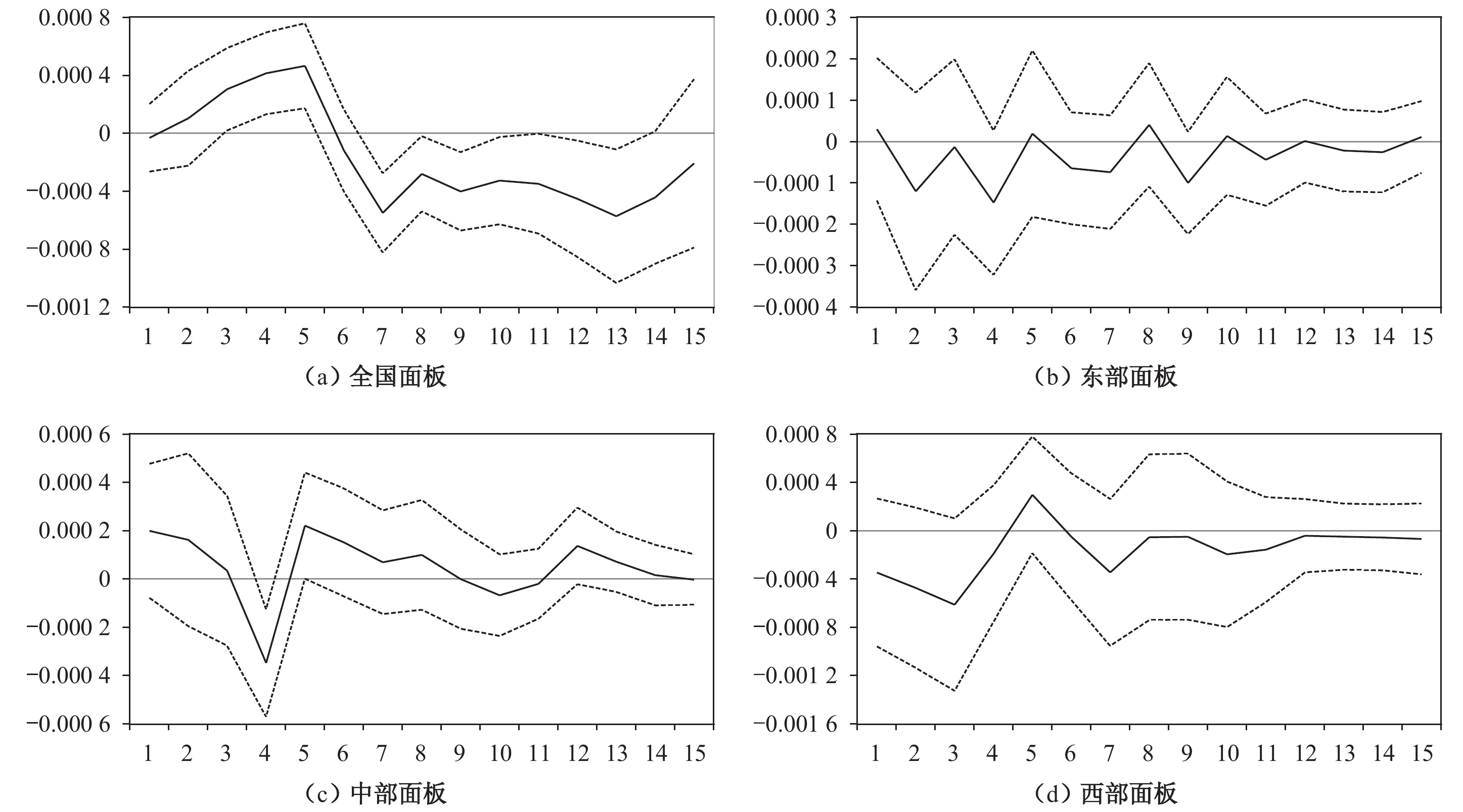﻿ 收入分配与政府税收:“国富”的一种解释
 上海财经大学学报2017, Vol. 19Issue (2): 13-260

#### 文章信息上海财经大学2017年19卷第2期

Li Zilian, Wang Aimin, Li Xiao

Income Distribution and Government Tax Revenues :An Explanation of China’s Wealthy Government

Journal of Shanghai University of Finance and Economics , 2017, 19(2): 13-26.

### 文章历史《上海财经大学学报》
2017第19卷第2期

1.江苏师范大学 商学院,江苏 徐州　221116;
2.江苏师范大学 科文学院,江苏 徐州　221116

①此小节主要思想来源于作者的博士学位论文（李子联,2012）,但有较大改动和完善.

 ${T_1} = \left( {{y_b} - {y_a} - \mu } \right)t$

 ${T_2} = \left( {{y_b} + m - {y_a} - \mu } \right)t$
 $\Delta T = {T_2} - {T_1} = mt$

 ${T_1} = \left( {{y_a} - {y_a} + \mu } \right)t + \left( {{y_b} - {y_a} + \mu } \right)t$

 ${T_2} = \left( {{y_b} + m - {y_a} + \mu } \right)t$
 $\Delta T = {T_2} - {T_1} = mt - \mu t > 0$

 $T_2' = \left( {{y_a} - m - {y_a} + \mu } \right)t + \left( {{y_b} + m - {y_a} + \mu } \right)t$
 $\Delta T = T_2' - {T_1} = - mt + mt = 0$

 ${T_1} = \left( {{y_a} - {y_a} + \mu } \right){t_1} + \left( {{y_b} - {y_a} + \mu } \right){t_1} + \left( {{y_c} - {y_a} + \mu } \right){t_1}$

 ${T_2} = \left( {{y_c} + 2m - {y_c} - \eta } \right){t_2} + \left( {{y_c} + \eta - {y_a} + \mu } \right){t_1}$
 $\Delta T = \left( {2m - \eta } \right){t_2} - \left( {2\mu - \eta } \right){t_1}$

TI为泰尔指数,用以衡量收入分配制度或分配方式的平等与否.不同于大多数文献所采用的基尼系数和城乡收入差距指标,我们拟运用泰尔指数来衡量收入分配制度的平等程度,不仅是因为这一指标更易计算和统计,更是因为它考虑了人口权重,因而能够更好地观测收入分配的差异.其计算公式是:若以yi y分别表示不同组别（包括城镇居民和农村居民两个组别,下同）的收入和总收入,以pi p分别表示不同组别的人口和总人口,则泰尔指数可以表示为:

 $TI = \mathop \sum \limits_{i = 1}^n \frac{{{y_i}}}{y}\left( {{\rm log}\frac{{{y_i}}}{y} - {\rm log}\frac{{{p_i}}}{p}} \right)$图 1 全国财政税收与收入分配的统计关系 数据来源:根据1979–2015年的《中国统计年鉴》整理计算而得.图 2 政府税收与收入分配不平等呈反向变化的省份 资料来源:根据1999–2015年各省份统计年鉴的相关数据整理计算而得.

 $\begin{array}{l}\Delta {\left( {ptax/GDP} \right)_t} = {\alpha _1}\Delta T{I_{t - 1}} + {\alpha _2}\Delta T{I_{t - 2}} + \ldots + {\alpha _4}\Delta T{I_{t - 4}} + {\beta _1}\Delta {\left( {ptax/GDP} \right)_{t - 1}}\\ + {\beta _2}\Delta {\left( {ptax/GDP} \right)_{t - 2}} + \ldots + {\beta _4}\Delta {\left( {ptax/GDP} \right)_{t - 4}} + C\end{array}$
 $\begin{array}{l}\Delta {\left( {tax/GDP} \right)_t} = {\alpha _1}\Delta T{I_{t - 1}} + {\alpha _2}\Delta T{I_{t - 2}} + \ldots + {\alpha _6}\Delta T{I_{t - 9}} + {\beta _1}\Delta {\left( {tax/GDP} \right)_{t - 1}} + {\beta _2}\Delta {\left( {tax/GDP} \right)_{t - 2}}\\+ \ldots + {\beta _6}\Delta {\left( {tax/GDP} \right)_{t - 9}} + C\end{array}$
 $\begin{array}{l}\Delta {\left( {ptax/GDP} \right)_{i,t}} = {\alpha _1}\Delta T{I_{i,t - 1}} + {\alpha _2}\Delta T{I_{i,t - 2}} + \ldots + {\alpha _n}\Delta T{I_{i,t - n}} + {\beta _1}\Delta {\left( {ptax/GDP} \right)_{i,t - 1}}\\ + {\beta _2}\Delta {\left( {ptax/GDP} \right)_{i,t - 2}} + \ldots + {\beta _n}\Delta {\left( {ptax/GDP} \right)_{i,t - n}} + C\end{array}$

①由于篇幅所限,各变量的平稳性检验表并未在文中展示,备索.图 3 全国时间序列下收入分配冲击税收的效应图 4 省际面板数据下收入分配冲击税收的效应

  李子联. 收入分配如何影响经济增长——一个基于需求视角的分析框架[J].财经科学,2011(5).  李子联.中国的收入不平等与经济增长[D].南京:南京大学,2012.  李子联,朱江丽. 收入分配与经济增长:中国经济增长模式的再解读[J]. 上海财经大学学报,2015,（4）.  莫亚琳, 张志超. 改革开放以来我国财政支出对收入分配影响的研究——基于城乡二元结构模型与面板数据的分析[J].经济体制改革,2011(2).  陶然, 刘明兴. 中国城乡收入差距,地方政府开支及财政自主[J].世界经济文汇,2007(2).  尹恒, 龚六堂, 邹恒甫. 收入分配不平等与经济增长:回到库兹涅茨假说[J].经济研究,2005(4).  Bowles S,Gintis H. Reciprocity,self-interest and the welfare state[J]. Nordic Journal of Political Economy,2000,26:33–53.  Dallinger U. Public support for redistribution:what explains cross-national differences?[J].Journal of European Social Policy,2010,20(4):333–349.  Iversen T, D Soskice. Electoral institutions and the politics of coalitions:Why some democracies redistribute more than others[J].The American Political Science Review,2006,100(2):165–181.  Jaeger M M. The effect of macroeconomic and social conditions on the demand for redistribution:A pseudo panel approach[J].Journal of European Social Policy,2013,23(2):149–163.  Kelly N J,Enns P K. Inequality and the dynamics of public opinion:The self-reinforcing link between economic inequality and mass preferences[J].American Journal of Political Science,2010,54(4):855–870.  Kenworthy L,McCall L,public opinion and redistribution[J]. Socio-Economic Review,2008,6(1):35–68.  Linos K, M West. Self-interest,social beliefs,and attitudes to redistribution:Re-addressing the issue of cross-national variation[J].European Sociological Review,2003,19(4):393–409.  Lübker M. Inequality and the demand for redistribution:Are the assumptions of the new growth theory valid?[J]. Socio-Economic Review,2007,5(1):117–148.  Meltzer A H,Richard S F. A rational theory of the size of government[J].Journal of Political Economy,1981,89(5):914–927.  Moene K O,Wallerstein M. Inequality,social insurance,and redistribution[J]. The American Political Science Review,2001,95(4):859–874.  Rehm P. Risks and redistribution:An individual-level analysis[J].Comparative Political Studies,2009,42(7):855–881.  Roberts K W S. Voting over income tax schedules[J].Journal of Public Economics,1977,8(3):329–340.  Romer T. Individual welfare,majority voting,and the properties of a linear income tax[J].Journal of Public Economics,1975,4(2):163–185.  Schmidt-Catran A W. Economic inequality and public demand for redistribution:Combining cross-sectional and longitudinal evidence[J].Socio-Economic Review,2016,14(1):119–140.
Income Distribution and Government Tax Revenues :An Explanation of China’s Wealthy Government
Li Zilian1, Wang Aimin1, Li Xiao2
1.School of Business,Jiangsu Normal University,Jiangsu Xuzhou 221116,China;
2.Kewen College,Jiangsu Normal University,Jiangsu Xuzhou 221116,China
Abstract: The degree of the richness in China’s government,which is measured by the share of government tax revenues in gross national income,is basically determined by the distribution model among residents. It is proved by some points as the follows:firstly,income inequality,the ration of different tax rates in the progressive rate institution and income threshold in different tax grades are important determinants of government tax revenue scale；secondly,the progressive tax institution and benefit sharing model of redistribution make the proportion of government tax revenues in the gross national income rise effectively,even if the income gap between residents expands or not,which is the reason for the formation of the wealthy government； lastly,spatial difference exists in the effect of income inequality on government tax revenues:the rise in the proportion of provincial governments in Central China is related to the expansion of income gap while the increase in provincial government tax revenues in Eastern China and Western China is due to the improvement of income distribution. Therefore,to deepen the reform of income distribution,we should not only raise the tax rates and the income threshold,but also continue to take the benefit-sharing redistribution to realize the constant rise in tax revenues and increasingly narrow the income gap.
Key words: income distribution     government tax revenue     benefit sharing